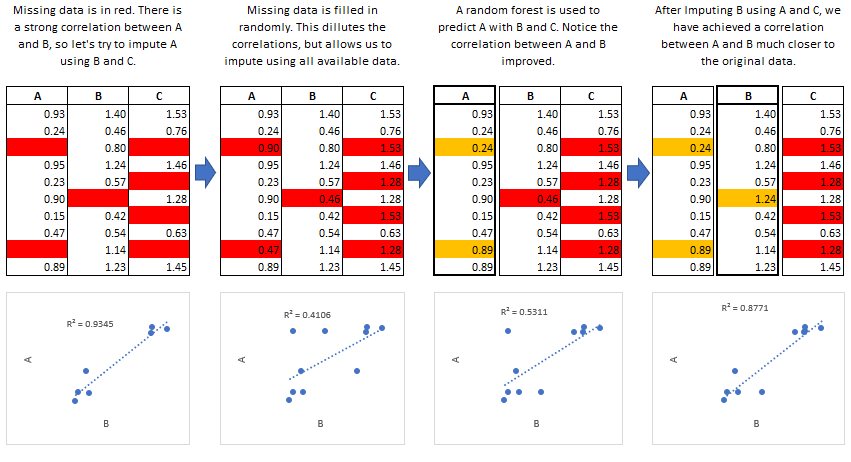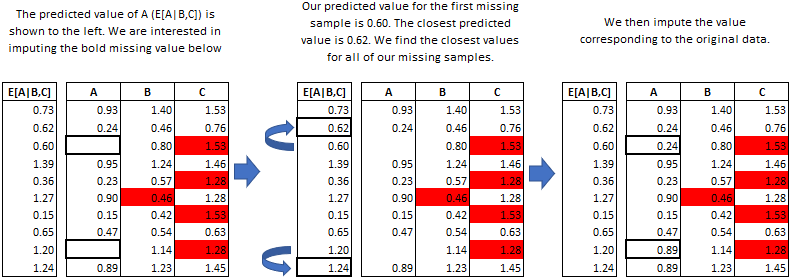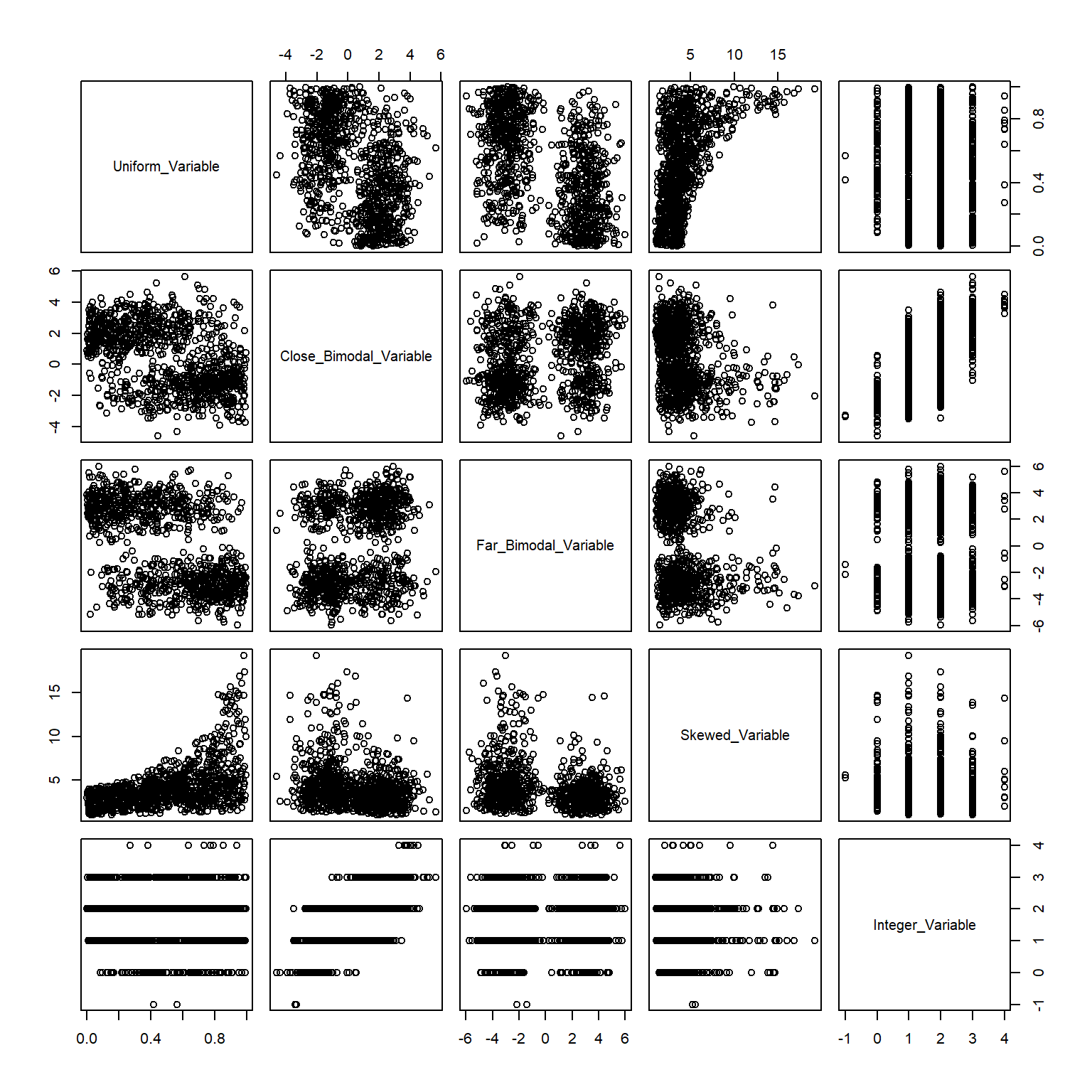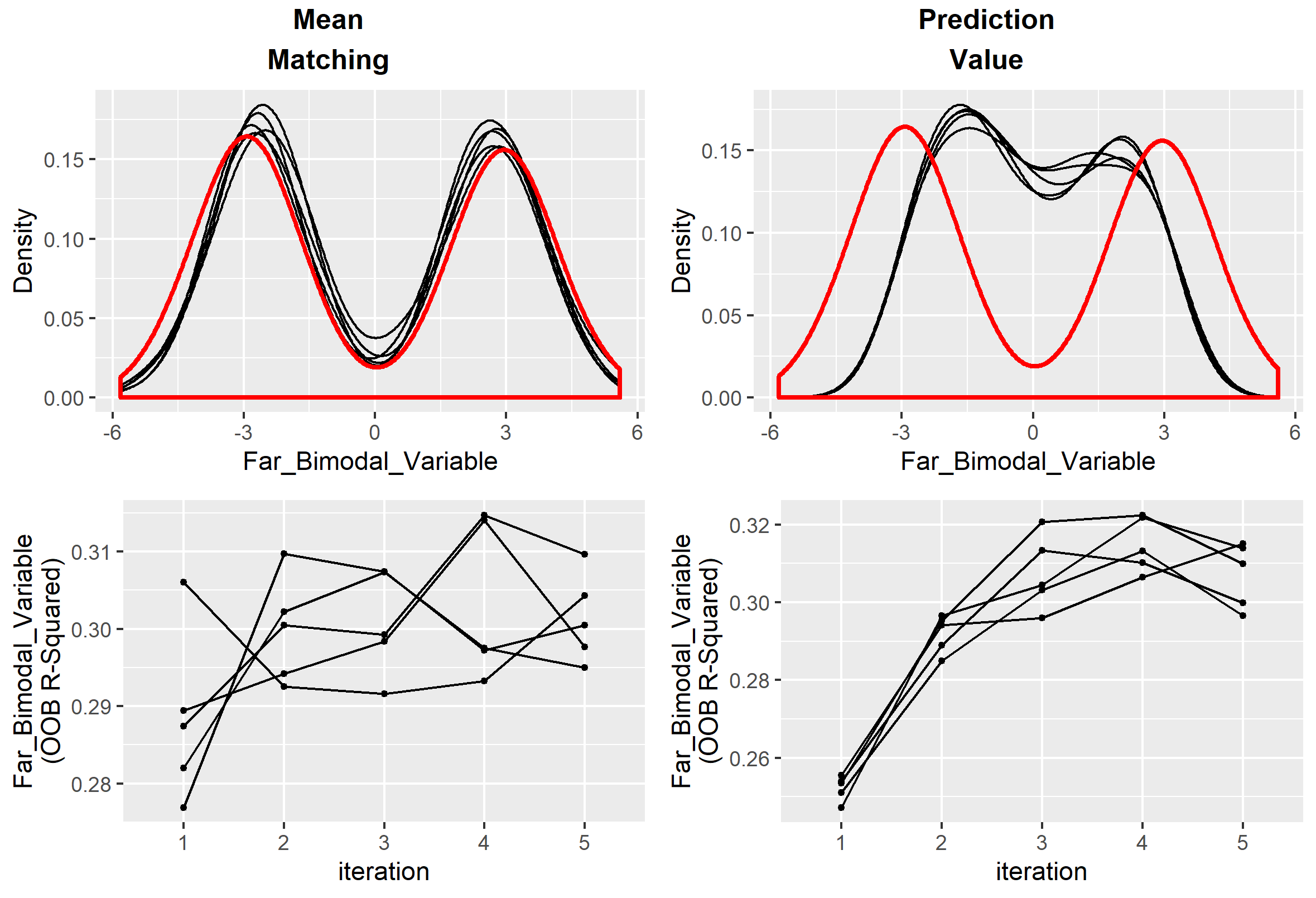## Introduction

Multiple Imputation by Chained Equations is a robust, informative method of dealing with missing data in datasets. The procedure ‘fills in’ (imputes) missing data in a dataset through an iterative series of predictive models. In each iteration, each specified variable in the dataset is imputed using the other variables in the dataset. These iterations should be run until it appears that convergence has been met.This process is continued until all specified variables have been imputed. Additional iterations can be run if it appears that the average imputed values have not converged, although no more than 5 iterations are usually necessary. The accuracy of the imputations will depend on the information density in the dataset. A dataset of completely independent variables with no correlation will not yield accurate imputations. There are diagnostic plots available in `miceRanger` which allow the user to determine how valid the imputations may be.

### Predictive Mean Matching

`miceRanger` can make use of a procedure called predictive mean matching (PMM) to select which values are imputed. PMM involves selecting a datapoint from the original, nonmissing data which has a predicted value close to the predicted value of the missing sample. The closest N (`meanMatchCandidates` parameter in `miceRanger()`) values are chosen as candidates, from which a value is chosen at random. Going into more detail from our example above, we see how this works in practice:This method is very useful if you have a variable which needs imputing which has any of the following characteristics:

• Multimodal
• Integer
• Skewed

### Effects of Mean Matching

As an example, let’s construct a dataset with some of the above characteristics:

``````library(data.table)
library(miceRanger)

# random uniform variable
nrws <- 1000
dat <- data.table(Uniform_Variable = runif(nrws))

# slightly bimodal variable correlated with Uniform_Variable
dat\$Close_Bimodal_Variable <- sapply(
dat\$Uniform_Variable
, function(x) sample(c(rnorm(1,-2),rnorm(1,2)),prob=c(x,1-x),size=1)
) + dat\$Uniform_Variable

# very bimodal variable correlated with Uniform_Variable
dat\$Far_Bimodal_Variable <- sapply(
dat\$Uniform_Variable
, function(x) sample(c(rnorm(1,-3),rnorm(1,3)),prob=c(x,1-x),size=1)
)

# Highly skewed variable correlated with Uniform_Variable
dat\$Skewed_Variable <- exp((dat\$Uniform_Variable*runif(nrws)*3)) + runif(nrws)*3

# Integer variable correlated with Close_Bimodal_Variable and Uniform_Variable
dat\$Integer_Variable <- round(dat\$Uniform_Variable + dat\$Close_Bimodal_Variable/3 + runif(nrws)*2)

# Ampute the data.
ampDat <- amputeData(dat,0.2)

# Plot the original data
plot(dat)``````We can see how our variables are distributed and correlated in the graph above. Now let’s run our imputation process twice, once using mean matching, and once using the model prediction.

``````mrMeanMatch <- miceRanger(ampDat,valueSelector = "meanMatch",verbose=FALSE)
mrModelOutput <- miceRanger(ampDat,valueSelector = "value",verbose=FALSE)``````

Let’s look at the effect on the different variables.

#### Bimodial VariableThe affect of mean matching on our imputations is immediately apparent. If we were only looking at model error, we may be inclined to use the Prediction Value, since it has a higher OOB R-Squared. However, we are left with imputations that do not match our original distribution, and therefore, do not behave like our original data.